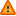FAQ/causation - CBU statistics WikiYou should trust both wikis because the password could be read by the particular administrators.

Clear message
location: FAQ / causation

# Reciprocal Links in a path model

It is usually not possible, in path analysis, to differentiate models with different causal links between a pair of variables. Suppose for example we had a variant of the model used in Sobel's test with a reciprocal link added between the mediator, M, and outcome, Y, as shown here. The direction of an arrow should be decided either through psychological theory or through temporal effects to see if mediation occurs prior to outcome.

In this instance the statistical model cannot distinguish between the direction of any arrow between M and Y. It can only test to see if any arrow, or association exists.

To see this consider what the arrow between M and Y used in Sobel's test represents. It represents the correlation between the residual from a simple regression of X (input) on M (call this res(M,X)) and the residual from a simple regression of X on Y (res(Y,X). Since r(res(M,X),res(Y,X)) = r(res(Y,X),res(M,X)) the value of the path coefficient will be the same for both directions that an arrow points between M and Y.

Estimating the M,Y given X correlations (e.g. using Preacher and Hayes SPSS macro (see Grad talks)) for the suicides data discussed in the grad talk where X is years in education and we have one model with M as standardized income and Y as standardized prestige score and one model with the M and Y variables swapped over we find The t-value for b(zprestige,zincome given years in education) = 4.1951 = the t-value for b(zincome,zprestige given years in education).

The regression coefficients are 0.8764 (se=0.2089) for Y=zincome,M=zprestige given X=education and 0.3968 (0.0946) for Y=prestige,M=zincome given X=education. The ratio of both the regression coefficients and their standard errors = 2.208. The differences in regression coefficients and standard errors is merely down to the differing variances of the residuals from simple regressions of zprestige predicted by education (zpe) and zincome predicted by education (zie). To see this we obtain the residuals whose variance ratio is 0.75922/0.51092 = 2.208 which is the scalar difference of the two models swapping the M and Y variable around. In other words mediation models (of form used for Sobel's test) switching M and Y are indistinguishable and the regression coefficients and their standard errors only differ due to the differing variances (and in particular the residuals from removing X) of M and Y.

To further prove the equivalance to simple regressions of the full (X,M,Y) model, as fitted by the SPSS macro, simple regressions, using zpe to predict zie and vice-versa, give very similar regression estimates and standard errors (0.876, (0.206); 0.397, (0.093) to the Preacher annd Hayes SPSS full model fit described above. The simple regressions each have a common t-value of 4.258 which agrees with Preacher and Hayes's 4.195 to 1 decimal place.

For more complex models than that tested by Sobel where one variable has a predictor as here changing the direction of a causation arrow will result in statistically different models with different overall fits and regression estimates. This is because the residuals, being correlated to obtain these regression coefficients, will be different depending which variable is mediating (M) and which is outcome (Y).